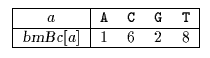Next: Reverse Factor algorithm Up: ESMAJ Prev: Smith algorithm

# Raita algorithm

Main features
• first compares the last pattern character, then the first and finally the middle one before actually comparing the others;
• performs the shifts like the Horspool algorithm;
• preprocessing phase in O(m+) time and O() space complexity;
• searching phase in O(mn) time complexity.
Description

Raita designed an algorithm which at each attempt first compares the last character of the pattern with the rightmost text character of the window, then if they match it compares the first character of the pattern with the leftmost text character of the window, then if they match it compares the middle character of the pattern with the middle text character of the window. And finally if they match it actually compares the other characters from the second to the last but one, possibly comparing again the middle character.

Raita observed that its algorithm had a good behaviour in practice when searching patterns in English texts and attributed these performance to the existence of character dependencies.
Smith made some more experiments and concluded that this phenomenon may rather be due to compiler effects.

The preprocessing phase of the Raita algorithm consists in computing the bad-character shift function (see chapter Boyer-Moore). It can be done in O(m+) time and O() space complexity.

The searching phase of the Raita algorithm has a quadratic worst case time complexity.

The C code

The function preBmBc is given chapter Boyer-Moore algorithm.

```void RAITA(char *x, int m, char *y, int n) {
int j, bmBc[ASIZE];
char c, firstCh, *secondCh, middleCh, lastCh;

/* Preprocessing */
preBmBc(x, m, bmBc);
firstCh = x;
secondCh = x + 1;
middleCh = x[m/2];
lastCh = x[m - 1];

/* Searching */
j = 0;
while (j <= n - m) {
c = y[j + m - 1];
if (lastCh == c && middleCh == y[j + m/2] &&
firstCh == y[j] &&
memcmp(secondCh, y + j + 1, m - 2) == 0)
OUTPUT(j);
j += bmBc[c];
}
}

```
The example

Preprocessing phasebmBc table used by Raita algorithm.

Searching phase

References
• RAITA T., 1992, Tuning the Boyer-Moore-Horspool string searching algorithm, Software - Practice & Experience, 22(10):879-884.
• SMITH, P.D., 1994, On tuning the Boyer-Moore-Horspool string searching algorithms, Software - Practice & Experience, 24(4):435-436.Next: Reverse Factor algorithm Up: ESMAJ Prev: Smith algorithm

e-mails: {Christian.Charras, Thierry.Lecroq }@laposte.net
Tue Jan 14 15:03:31 MET 1997
Tue Jan 14 15:03:31 MET 1997